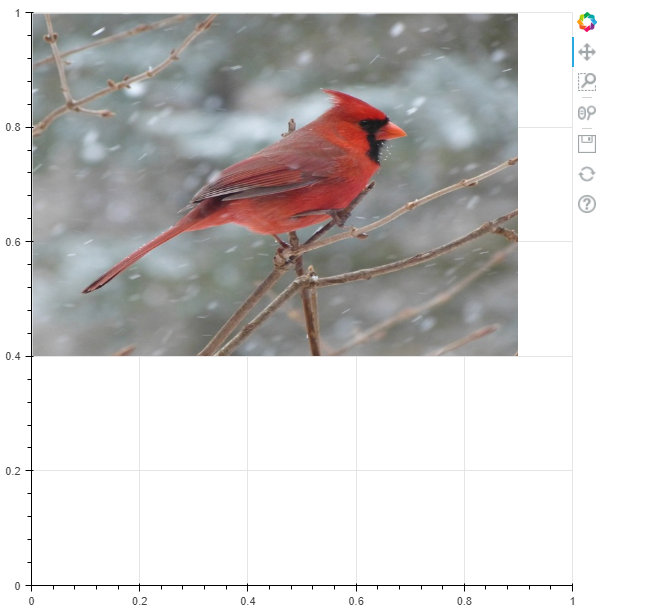How to work with images in Bokeh (Python)?

MatplotlibPythonData Visualization

To work with images in Bokeh, use image_url() method and pass a list of images.

Steps

• Configure the default output state to generate output saved to a file when :func:'show' is called.
• Create a new Figure for plotting.
• Render the images loaded from the given URLs.
• Immediately display a Bokeh object or application.

Example

from bokeh.plotting import figure, show, output_file

output_file('image.html')

p = figure(x_range=(0, 1), y_range=(0, 1))
p.image_url(url=['bird.jpg'], x=0, y=1, w=0.8, h=0.6)
show(p)

Output